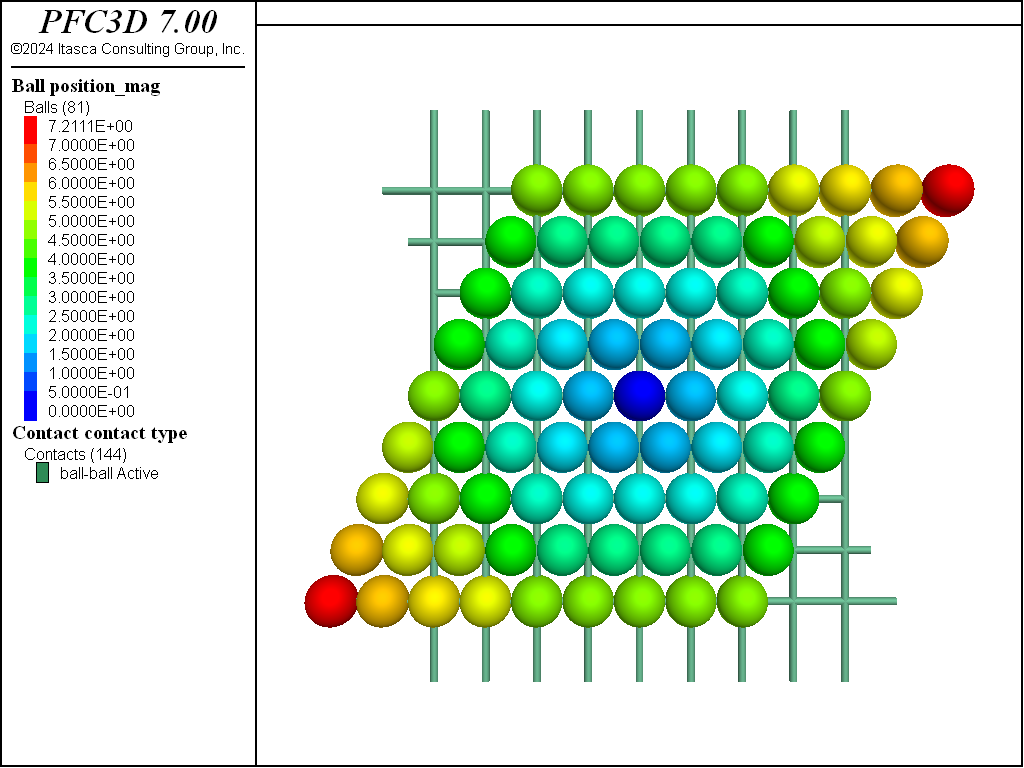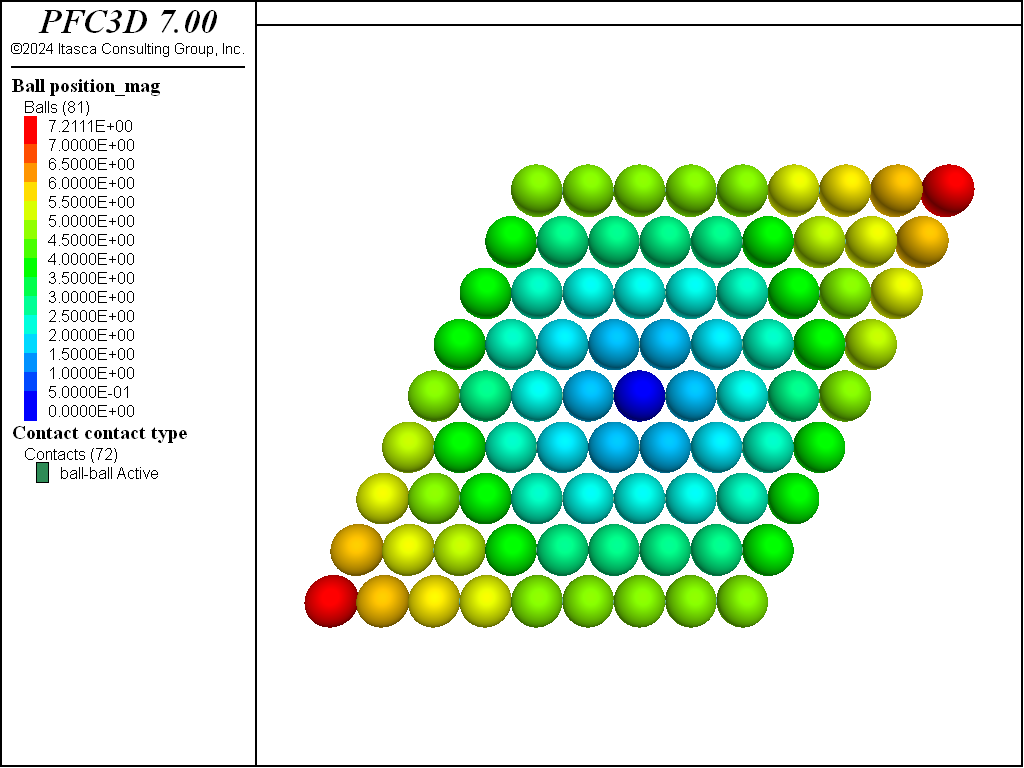# ball attribute command

Syntax

ball attribute keyword ... <range>

Primary keywords:

Set the value of ball attributes. This command is a synonym for the ball initialize command. Individual attributes can be listed with the ball list command and all attributes can be visualized.

Note

• Modification of the ball geometry will fail if the resulting ball would not fall entirely within the model domain.
• Ball attributes are characteristics of the balls such as position or size. These are distinct from ball properties (assigned with the ball property command) that are surface properties of the balls that are used to fill contact model properties.
damp f [modblock]

Ball local damping coefficient where f ≥ 0. By default, f = 0.

density f [modblock]

Ball density, where f > 0. By default, ball density is set to zero upon creation of a ball; a non-zero value is required for PFC to properly compute mass properties and integrate the equation of motion for the ball. An exception is thrown if a ball with zero density exists in the model when cycling starts.

displacement v [modblock]

Accumulated ball displacement vector as a result of cycling.

displacement-x f [modblock]

The $$x$$-component of the accumulated ball displacement as a result of cycling.

displacement-y f [modblock]

The $$y$$-component of the accumulated ball displacement as a result of cycling.

displacement-z f (3D ONLY) [modblock]

The $$z$$-component of the accumulated ball displacement as a result of cycling.

euler v (3D ONLY) [modblock]

Current orientation of Euler angles following the X,Y,Z convention (e.g., rotation about the $$x$$-axis followed by rotation about the y’-axis followed by rotation about the z’‘-axis) in degrees. The orientation is updated only when orientation tracking has been enabled (see model orientation-tracking command). When active, the current ball orientation can be visualized.

euler-x f (3D ONLY) [modblock]

The $$x$$-euler angle (in degrees) of the current ball orientation. See the euler keyword for further details.

euler-y f (3D ONLY) [modblock]

The $$y$$-euler angle (in degrees) of the current ball orientation. See the euler keyword for further details.

euler-z f (3D ONLY) [modblock]

The $$z$$-euler angle (in degrees) of the current ball orientation. See the euler keyword for further details.

force-applied v [modblock]

Force applied to balls.

force-applied-x f [modblock]

The $$x$$-component of the force applied to balls.

force-applied-y f [modblock]

The $$y$$-component of the force applied to balls.

force-applied-z f (3D ONLY) [modblock]

The $$z$$-component of the force applied to balls.

force-contact v [modblock]

Sum of the contact force accumulated to the balls during the previous force-displacement update. This value will be modified during the next force-displacement update.

force-contact-x f [modblock]

The $$x$$-component of the contact force.

force-contact-y f [modblock]

The $$y$$-component of the contact force.

force-contact-z f (3D ONLY) [modblock]

The $$z$$-component of the contact force.

fragment i

Fragment ID (see “Fragment”).

moment-applied fx fy fz (y- and z- components are 3D ONLY) [modblock]

Moment applied to balls.

moment-applied-x f (3D ONLY) [modblock]

The $$x$$-component of the moment applied to balls.

moment-applied-y f (3D ONLY) [modblock]

The $$y$$-component of the moment applied to balls.

moment-applied-z f (3D ONLY) [modblock]

The $$z$$-component of the moment applied to balls.

moment-contact fx fy fz (y- and z- components are 3D ONLY) [modblock]

Sum of the contact moments accumulated to the balls during the previous force-displacement update. This value will be modified during the next force-displacement update.

moment-contact-x f (3D ONLY) [modblock]

The $$x$$-component of the contact moment.

moment-contact-y f (3D ONLY) [modblock]

The $$y$$-component of the contact moment.

moment-contact-z f (3D ONLY) [modblock]

The $$z$$-component of the contact moment.

position v [modblock]

Location of ball centroid. Note that the model should be cleaned (see the model clean command) after this command is issued to ensure that potential contacts with neighboring pieces are updated.

position-x f [modblock]

The $$x$$-component of the location of ball centroids.

position-y f [modblock]

The $$y$$-component of the location of ball centroids.

position-z f (3D ONLY) [modblock]

The $$z$$-component of the location of ball centroids.

Ball radius where f > 0. A positive radius is required at ball creation (using the ball create, ball generate, or ball distribute commands). Ball radii can be subsequently modified using this command. Note that the model should be cleaned (see the model clean command) after this command is issued to ensure that potential contacts with neighboring pieces are updated.

rotation f (2D ONLY) [modblock]

Current ball orientation. The orientation is updated only when orientation tracking has been enabled (see model orientation-tracking command).

spin fx fy fz (y- and z- components are 3D ONLY) [modblock]

Ball angular velocity.

spin-x f (3D ONLY) [modblock]

The $$x$$-component of the ball angular velocity in radians.

spin-y f (3D ONLY) [modblock]

The $$y$$-component of the ball angular velocity in radians.

spin-z f (3D ONLY) [modblock]

The $$z$$-component of the ball angular velocity in radians.

velocity v [modblock]

Ball translational velocity vector.

velocity-x f [modblock]

The $$x$$-component of the ball velocity.

velocity-y f [modblock]

The $$y$$-component of the ball velocity.

velocity-z f (3D ONLY) [modblock]

The $$z$$-component of the ball velocity.

ball attribute Keyword Block

Add f to the existing value.

Apply a linear gradient in each of the axes directions starting at the origin.

Apply a linear gradient in the $$x$$-direction starting at the origin.

Apply a linear gradient in the $$y$$-direction starting at the origin.

Apply a linear gradient in the $$z$$-direction starting at the origin.

multiply f

Multiply the existing value by f.

replace f

Replace the existing value with f.

Usage Example

Be aware of the distinction between attributes and properties! The tutorial example “Attributes and Properties” discusses this issue in detail.

Typical Usage

Set the Ball Density

The most typical usage of the ball attribute command is to set the density (and local damping coefficient if pertinent) of previously created balls, as illustrated in the following example. Note that a non-zero density must be specified for each ball of the system, otherwise PFC will throw an exception and stop at the beginning of the cycle sequence.

; setup model domain
model domain extent -10 10
model large-strain on

; select default contact model and its properties
contact cmat default model linear property kn 1e6

; generate a random assembly of balls in a box
model random 10001
wall generate box -5 5 one-wall
ball generate id 1 500 box -4.5 4.5 radius 0.5

; assign density and local damping coefficient to existing balls
ball attribute density 1000.0 damp 0.7

; set gravity and solve the system
model gravity 10.0
model solve


Set Initial Conditions

Another common usage of the ball attribute command is to set initial conditions. In the following example, a row of balls is generated, then a selected range of balls are assigned a non-zero velocity while the remaining balls are assigned a non-zero applied force. Note that since velocity is a quantity that is updated every timestep during resolution of the equations of motion, it evolves with time as the system is cycled. On the other hand, the applied force is never modified by PFC.

model large-strain on
; setup model domain
model domain extent -10 10 condition destroy

; select default contact model and its properties
contact cmat default model linear property kn 1e6 dp_nratio 0.2

; generate a random assembly of balls in a box
model random 10001
wall generate box -5 5 one-wall
ball generate id 1 100 box -4.5 4.5 0.0 0.0 radius 0.5 cubic
; assign density and local damping coefficient to existing balls
ball attribute density 1000.0

; set the velocity attribute for a selected range of balls
; note that ball velocitied will be updated during cycling
ball attribute velocity (0.0,0.0,-1.0) range position-x -4.5 0.0

; set the applied force attribute of remaining balls
; since applied force is never updated during the cycling, this
; value will remain constant
ball attribute force-applied (0.0,0.0,-1.0) range position-x -4.5 0.0 not

; perform cycles
model cycle 10000


The following code will set the velocity and fix its value for a selected range of balls. As a result, these balls do exit the model, although they interact with the box wall.

model large-strain on
; set velocity for all the balls, and fix the x-velocity component
; for a selected range
ball attribute velocity (0.5,0.0,0.0)
ball fix velocity-x range position-x -4.5 0.0

; perform cycles - the balls with fixed velocities exit the domain
model cycle 5000


Modifying the System Geometry

Some attributes may be used to modify the geometry of the system. This is the case for position and radius, for instance. The following example demonstrates this possibility. A sheet of balls is first generated on a square lattice (Figure 2 shows the initial system; balls and contacts are plotted).

; setup model domain
model domain extent -10 10 condition destroy
model large-strain on

; select default contact model and its properties
contact cmat default model linear property kn 1e6 dp_nratio 0.2

; generate a cubic plane of balls in a box
; also clean the model to detect contacts
model random 10001
ball generate id 1 100 box -4.0 4.0 0.0 0.0 -4.0 4.0 radius 0.5 cubic
model clean


The following command modifies ball positions, resulting in the system shown on Figure 3. Note that the contact list is not updated automatically. Although it will be updated at the beginning of the next cycle sequence, this may be problematic if the user relies on this information to perform some operation before any further cycles are performed. In such a case, the model needs to be cleaned.

; modify ball x-positionsIssuing the model clean command does update the contact list (see Figure 4).
; clean the model to update the contact list